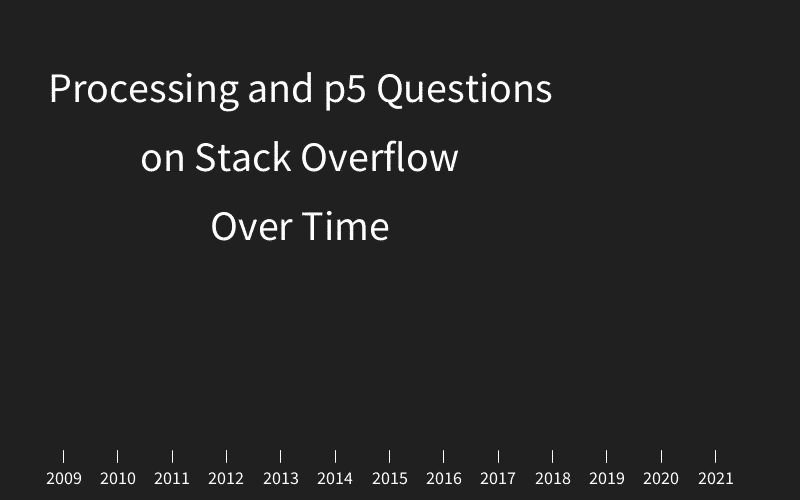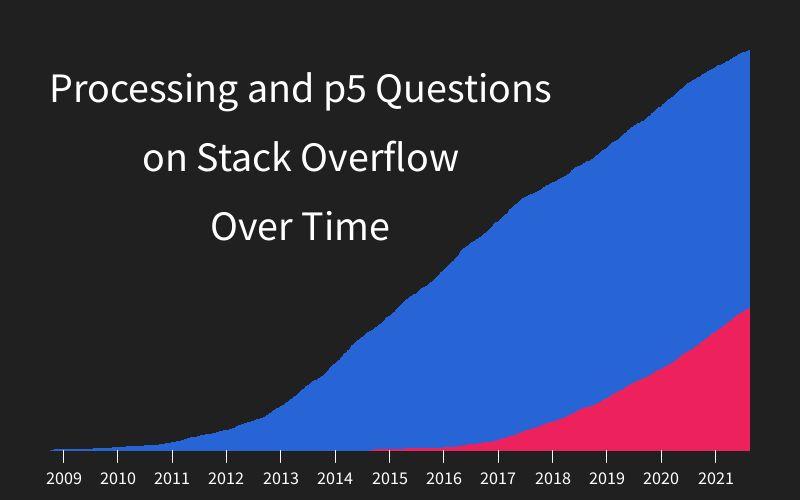# Stack Overflow Data Visualization

example processing

To celebrate Processing’s 20th anniversary, I decided to use Processing to visualize how many questions have been asked in the Processing and p5.js tags on Stack Overflow.

Answering questions on Stack Overflow was a big part of how I became involved in the Processing and p5.js communities, so it was cool to use Processing itself to visualize this journey.

I split this into three parts:

Processing 4.0 was released just a few days before I created this data visualization, so I was excited to try it out!

# Part 2: Massage the Data

“Massaging the data” means taking data that’s in one format, and converting it to a different format that’s easier to work with.

In this case, I fetched data from the questions endpoint of the Stack Overflow API and converted it to an array of timestamps.

The converter code ended up looking like this:

``````import java.io.InputStreamReader;
import java.nio.charset.StandardCharsets;
import java.net.HttpURLConnection;
import java.net.URL;
import java.util.zip.GZIPInputStream;
import java.util.stream.Collectors;

void setup() {
try {
JSONArray dates = new JSONArray();

boolean hasMore = true;
int currentPage = 1;

while (hasMore) {
println("Fetching page: " + currentPage);

String text = getUrlContent(currentPage);
JSONObject top = parseJSONObject(text);
JSONArray itemsArray = top.getJSONArray("items");
ArrayList<Integer> pageList = convertJsonArrayToDates(itemsArray);
for (int date : pageList) {
dates.append(date);
}

hasMore = top.getBoolean("has_more");
currentPage++;
}

println(dates.size());

saveJSONArray(dates, "processing-questions.json");
}
catch(Exception e) {
e.printStackTrace();
}
}

ArrayList<Integer> convertJsonArrayToDates(JSONArray input) {
ArrayList<Integer> list = new ArrayList<>();

for (int i = 0; i < input.size(); i++) {
int date = input.getJSONObject(i).getInt("creation_date");
}

return list;
}

String getUrlContent(int currentPage) throws Exception {

String href = "https://api.stackexchange.com/2.3/questions?pagesize=100&order=asc&sort=creation&tagged=processing&site=stackoverflow";
href += "&page=" + currentPage;

URL url = new URL(href);
HttpURLConnection connection = (HttpURLConnection) url.openConnection();
connection.setRequestProperty("Accept-Encoding", "DEFLATE");

.lines()
.collect(Collectors.joining("\n"));

return text;
}
``````

This “middle step” of massaging the data is very common in projects like this, but it’s not often shown. I got pretty sidetracked debugging a problem related to encoding, but I eventually figured it out. The code might not be pretty, but it works!

# Part 3: Visualize the Data

Now that I had the data file(s) in a format that I could work with, I wrote code that used the data to draw a chart. The code ended up looking like this:

``````JSONArray processingQuestions;
JSONArray p5Questions;

int currentProcessingIndex = 1;
int currentP5Index = 1;

PGraphics processingPG;
PGraphics p5PG;

int firstDate;
int lastDate;
float totalDuration;

float border = 50;
float chartXPixels;
float chartYPixels;
float chartWidthPixels;
float chartHeightPixels;

float currentProcessingLineHeight = 0;
float currentP5LineHeight = 0;
float oneQuestionHeight;

HashMap<Integer, String> labelMap = new HashMap<>();

void setup() {
size(800, 500);
processingPG = createGraphics(width, height);
p5PG = createGraphics(width, height);

textAlign(CENTER, CENTER);

firstDate = processingQuestions.getInt(0);
lastDate = max(processingQuestions.getInt(processingQuestions.size() - 1),
p5Questions.getInt(p5Questions.size() - 1));

totalDuration = lastDate - firstDate;

chartXPixels = border;
chartYPixels = border;
chartWidthPixels = width - border * 2;
chartHeightPixels = height - border * 2;
oneQuestionHeight = chartHeightPixels / processingQuestions.size();

labelMap.put(1230796800, "2009");
labelMap.put(1262332800, "2010");
labelMap.put(1293868800, "2011");
labelMap.put(1325404800, "2012");
labelMap.put(1357027200, "2013");
labelMap.put(1388563200, "2014");
labelMap.put(1420099200, "2015");
labelMap.put(1451635200, "2016");
labelMap.put(1483257600, "2017");
labelMap.put(1514793600, "2018");
labelMap.put(1546329600, "2019");
labelMap.put(1577865600, "2020");
labelMap.put(1609488000, "2021");
}

void draw() {
background(32);
image(processingPG, 0, 0);
image(p5PG, 0, 0);

fill(255);
textSize(42);
text("Processing and p5 Questions\non Stack Overflow\nOver Time", 300, 150);

processingPG.beginDraw();
p5PG.beginDraw();
for (int i = 0; i < 10; i++) {
int currentProcessingDate = currentProcessingIndex < processingQuestions.size() ?
processingQuestions.getInt(currentProcessingIndex) : 0;
int currentP5Date = currentP5Index < p5Questions.size() ?
p5Questions.getInt(currentP5Index) : 0;

if ((currentProcessingDate > 0 && currentProcessingDate < currentP5Date)
|| currentP5Date == 0) {
stepProcessing();
} else {
stepP5();
}

if (currentProcessingIndex >= processingQuestions.size() &&
currentP5Index >= p5Questions.size()) {
println("Done!");
noLoop();
break;
}
}
processingPG.endDraw();
p5PG.endDraw();

textSize(18);
for (Integer date : labelMap.keySet()) {
PVector labelPoint = getPoint(date, 0);

stroke(255);
line(labelPoint.x, height - border,
labelPoint.x, height - border * .75);

fill(255);
text(labelMap.get(date), labelPoint.x, height - border / 2);
}

//saveFrame("f/#####.png");
}

void stepProcessing() {
int prevDate =  processingQuestions.getInt(currentProcessingIndex - 1);
int currentDate = processingQuestions.getInt(currentProcessingIndex);

PVector prevPoint = getPoint(prevDate, currentProcessingLineHeight);
PVector currentPoint = getPoint(currentDate, currentProcessingLineHeight);

processingPG.stroke(39, 101, 214);
processingPG.fill(39, 101, 214);
prevPoint.x, height - border,
prevPoint.x, prevPoint.y,
currentPoint.x, currentPoint.y,
currentPoint.x, height-border
);

currentProcessingLineHeight += oneQuestionHeight;

currentProcessingIndex++;
}

void stepP5() {
int prevDate =  p5Questions.getInt(currentP5Index - 1);
int currentDate = p5Questions.getInt(currentP5Index);

PVector prevPoint = getPoint(prevDate, currentP5LineHeight);
PVector currentPoint = getPoint(currentDate, currentP5LineHeight);

p5PG.stroke(237, 34, 93);
p5PG.fill(237, 34, 93);
prevPoint.x, height - border,
prevPoint.x, prevPoint.y,
currentPoint.x, currentPoint.y,
currentPoint.x, height-border
);

currentP5LineHeight += oneQuestionHeight;

currentP5Index++;
}

PVector getPoint(int date, float currentLineHeight) {
float currentDurationFromFirstDate = date - firstDate;
float currentPercent = currentDurationFromFirstDate / totalDuration;

float x = lerp(chartXPixels, width - border, currentPercent);
float y = height - border - currentLineHeight;

return new PVector(x, y);
}
``````

Some of this got a little messy when I decided to visualize p5.js data in addition to Processing data, but the idea should apply to pretty much any data visualization you implement: first you convert the data into a format you can work with, and then you write code that shows a graphical representation of that data.

In my case that’s a chart, but it could be anything you imagine!

# ResultOne thing I like about this project is that it shows “the middle step” of massaging the data. This is a common and crucial step in this kind of project, but it’s almost never shown. But this “middle step” was built right into Processing’s DNA from the start:

Happy birthday Processing!

# Remix Ideas

• Visualize other tags on Stack Overflow: how does Java compare to JavaScript?
• Visualize not just questions, but answers as well. Can you surface questions that still need answers?
• Show how different users have answered questions over time. When will George take back his title as Processing Answer Champion?